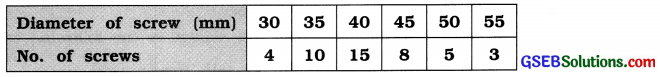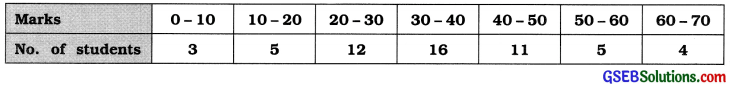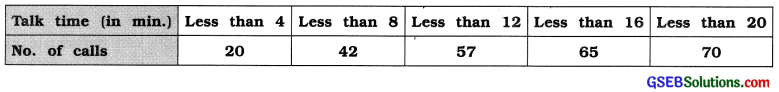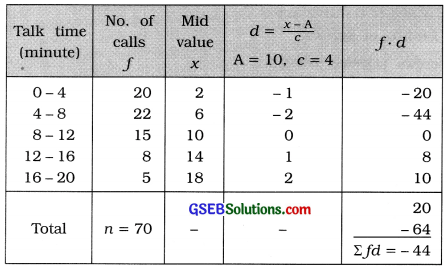# GSEB Solutions Class 11 Statistics Chapter 3 Measures of Central Tendency Ex 3.1

Gujarat Board Statistics Class 11 GSEB Solutions Chapter 3 Measures of Central Tendency Ex 3.1 Textbook Exercise Questions and Answers.

## Gujarat Board Textbook Solutions Class 11 Statistics Chapter 3 Measures of Central Tendency Ex 3.1

Question 1.
The weekly growths (in cm) in saplings grown in a nursery are:
1.0, 3.2, 1.4, 1.9, 2.4, 1.6, 1.4, 2.1, 1.3, 1.5 Find the mean growth.
Here, n = 10; x = weekly growth (in cm) of plant

Mean of growth of plant:
x̄ = $$\frac{\Sigma x}{n}$$
= $$\frac{1.0+3.2+1.4+1.9+2.4+1.6+1.4+2.1+1.3+1.5}{10}$$
= $$\frac{17.8}{10}$$
= 1.78 cm

Question 2.
The mean age of 4 participants in a relay race was calculated to be 24 years. Later it was found that one of the participant’s age was actually 27 years, which was wrongly recorded as 25 years. If there is rule wherein it is not possible to participate in this race if the mean age is more than 25 years, can they participate in this race even after the correction of age?
Here, Mean of age of 4 participants = 24 years
∴ Sum of age of 4 participants = 4 × 24 = 96 years

The age of one participants was taken 25 years instead of 27 years.
∴ True sum of age of 4 participants = 96 – 25 + 27 = 98 years
∴ Correct mean of age of 4 participants = $$\frac{98}{4}$$ = 24.5 years
If the age of one participant is more than 25 years, he cannot participate in the race.
The mean after the correction of age is 24.5 years. Therfore, a participants can participate in the race.Question 3.
The following table gives the diameters (in mm) of different screws selected from a large consignment. Find the mean diameter.Here, x = Diameter (in mm) of screw; f = No. of screws.
Therefore, the total number of screws selected n = Σf

Calculation of mean is shown in the following table :

 Diameter of the screw (mm) x No. of screws f f. x 30 4 120 35 10 350 40 15 600 45 8 360 50 5 250 55 3 165 Total n = 45 Σfx = 1845

Mean of diameter of screw:
x̄ = $$\frac{\Sigma f x}{n}$$
= $$\frac{1845}{45}$$
= 41 mm

Question 4.
The marks in a test for a group of students are as follows. Find the mean marks of these students.The given frequency distribution is of equal length. Therefore, taking d = $$\frac{x-\mathrm{A}}{c}$$, we calculate mean by short cut method. We prepare the calculation table as follows :Mean of marks of students:
x̄ = A + $$\frac{\sum f d}{n}$$ × c
Putting A = 35, Σfd = 2, n = 56 and c=10 in the formula,
x̄ = 35 + $$\frac{2}{562}$$ × c
x̄ = 35 + $$\frac{2}{562}$$
∴ x̄ = 35 + 0.36
∴ x̄ = 35.36 marks

Question 5.
The following information is available on the talk time (in min.) noted for 70 calls of a certain mobile phone user. Find the mean talk time.The given frequency distribution is ‘less than’ type cumulative frequency distribution. The difference between two successive upper boundary points is 4.

Therefore, class length c = 4 and we get lower boundary point of initial class as 0.
We find frequency of each class from the given cumulative frequency.

The original frequency distribution and the calculation of mean are shown in the following table :Mean of talk time;
x̄ = A + $$\frac{\sum f d}{n}$$ × c
Putting A = 10, Σfd = – 44, n = 70 and c = 4 in the formula,
x̄ = 10 – $$\frac{44}{70}$$ × 4
∴ x̄ = 10 – $$\frac{176}{70}$$
∴ x̄ = 10 – 2.51
∴ x̄ = 7.49 minutes
Hence, mean of talk time = 7.49 minutes.Question 6.
The information of profits (in lakh ₹) of 50 firms is given below. Find mean profit.The given frequency distribution is of equal length. Therefore, taking d = $$\frac{x-\mathrm{A}}{c}$$, we calculate mean by short cut method. The calculation table is prepared as follows:Mean of Profit:
x̄ = A + $$\frac{\sum f d}{n}$$ × c
Putting A = 17.5. Σfd = 9, n = 50 and c = 7 In the formula,
= 17.5 + $$\frac{9}{50}$$ × 7
= 17.5 + $$\frac{63}{50}$$
= 17.5 + 1.26
= ₹18.76 lakh
Hence, mean of profit Is ₹ 18.76 lakh.

Question 7.
The distribution of demand of an item on different days Is as follows. Find the mean demand.x̄ = A + $$\frac{\sum f d}{n}$$ × c
x̄ = 29.5 + $$\frac{189}{90}$$ × 5
∴ x̄ = 29.5 + $$\frac{945}{90}$$